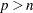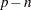Shared Concepts and Topics
 Lag Effects

EFFECT name=LAG (variable / lag-options) ;

A lag effect is a classification effect for the CLASS variable that is given after the keyword LAG. A lag effect is used to represent the effect of a previous value of the lagged variable when there is some inherent ordering of the observations of this variable. A typical example where lag effects are useful is a study in which different subjects are given sequences of treatments and you want to investigate whether the treatment in the previous period is important in understanding the outcome in the current period. You can do this by including a lagged treatment effect in your model.

The precise definition of a LAG effect depends on a subdivision of the data into disjoint subsets, often referred to as "subjects," and an ordering into units called "periods" of the observations within a subject. For an observation that belongs to a given subject and at a given period, the design matrix columns of the lagged variable are the usual design matrix columns of that variable except for the observation at the preceding period for that subject. Observations at the initial period do not have a preceding value, and so the design matrix columns of the lag effect for these observations are set to zero. You can also define lag effects where the number of periods that are lagged is greater than one. If the number of periods that are lagged is, then the design matrix columns of observations in periods less than or equal toare set to zero. The design matrix columns that correspond to a subject at period, where, are the usual design matrix columns of the lagged variable for that subject at period.

A convenient way to represent the organization of observations into subjects and periods is to form the lag design matrix. The rows and columns of this matrix correspond to the subjects and periods respectively. The lag design matrix entry is the treatment for the corresponding subject and period. In a valid lag design there is at most one observation for a given period and subject. For example, the following set of treatments by subject and period form a valid lag design:

```   Subject  Period   Treatment

Sheila      1        B
Joey        1        A
Athena      1        A
Gelindo     1        A
Sheila      2        C
Joey        2        A
Athena      2        .
Gelindo     2        B
Sheila      3        B
Joey        3        C
Athena      3        A
Gelindo     3        B

```

The associated lag design matrix is

```
--Period---
Subject    1    2    3

Athena     A         A
Gelindo    A    B    B
Joey       A    A    C
Sheila     B    C    B

```

Note that the subject Athena did not receive a treatment at period 2, and so the corresponding entry in the lag design matrix is missing. You can define a lag effect for this lag design with the following statements:

``` CLASS treatment;
EFFECT Lag = LAG( treatment / WITHIN=subject PERIOD=period);
```

When GLM coding is used for the CLASS variable treatment, the design matrix columns Lag_A, Lag_B, and Lag_C for the constructed effect Lag are as follows:

```   Subject  period  treatment  Lag_A  Lag_B  Lag_C

Athena      1        A        0      0      0
Athena      2                 1      0      0
Athena      3        A        .      .      .
Gelindo     1        A        0      0      0
Gelindo     2        B        1      0      0
Gelindo     3        B        0      1      0
Joey        1        A        0      0      0
Joey        2        A        1      0      0
Joey        3        C        1      0      0
Sheila      1        B        0      0      0
Sheila      2        C        0      1      0
Sheila      3        B        0      0      1

```

The design matrix columns for each subject at period 1 are all zero because there are no lagged observations for period 1. You can also see that the design matrix columns at period 3 for subject Athena are missing because Athena did not receive a treatment at period 2. Nevertheless, the design matrix columns for Athena at period 2 are nonmissing and correspond to the treatment "A" that she received in period 1.

The following lag-options are required:
PERIOD=variable

specifies the period variable of the LAG design. The number of periods is the number of unique formatted values of the PERIOD= variable, and the ordering of the period is formed by sorting these formatted values in ascending order. You must specify a PERIOD= variable.

WITHIN=(variables)
WITHIN=variable

specifies a variable (or a list of variables within parentheses) that defines the subject grouping of the lag design. If there is only one WITHIN= variable, then the parentheses are not required. Each subject is defined by the unique set of formatted values of the variables in the WITHIN= list. The subjects are sorted in ascending lexicographic order. You must specify a WITHIN= variable.

You can also specify the following lag-options:
DESIGNROLE=variable

specifies a numeric variable that is used to subset observations into a fitting group in which the value of the DESIGNROW= variable is nonzero and a second group in which the value of the specified variable is zero. The observations in the fitting group are used to form the LAG design matrix that is used in fitting the model. The LAG design that corresponds to the non-fitting group is used when scoring observations in the input data set that do not belong to the fitting group. This option is useful when you want to obtain predicted values in an output data set for observations that are not used in fitting the model. If you do not specify a DESIGNROLE= variable, then all observations are assigned to the fitting group.

DETAILS

requests a table that shows the lag design matrix of the lag effect.

NLAG= n

specifies the number of lags. By default NLAG=1.Previous Page | Next Page | Top of Page